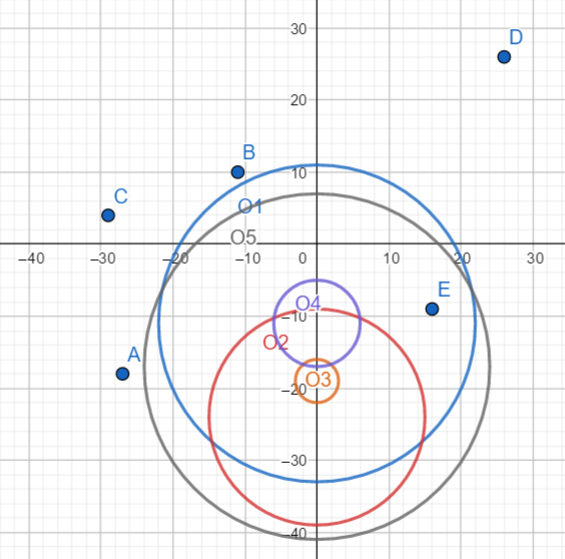# #553. 【UNR #4】己酸集合

### 输出格式

$Q$ 行，第 $i$ 行一个整数表示第 $i$ 次询问的答案。

### 样例一

#### input

5 5
-27 -18
-11 10
-29 4
26 26
16 -9
-11 22
-24 15
-19 3
-11 6
-17 24



#### output

1
0
0
0
1



#### explanation### 数据范围

$2$$\le 12000$$\le 5 \times 10^5$$30 3$$\le 4000$$\le 10^6$$20$
$4$$\le 7000$$20$
$5$$\le 12000$$15$